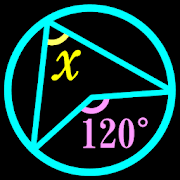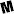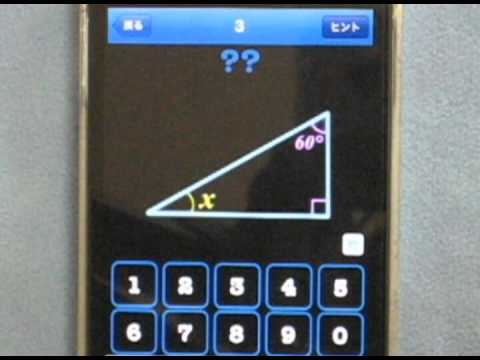# Find Angles! - Math questionsMature 17+
1,915Find Angles!
Once you have completed the basic questions from Stage 1 to Stage 4,
try to solve the challenging questions on the Final Stage!

If you can't solve a question, you can check the hint of the question or ask the answer to your friends via Twitter.

'Angles' contains following contents:
---
-The sum of the Angles of a triangle
-Exterior angle of a triangle
-Isosceles triangle
-Right-angled triangle

-Vertical angle
-Corresponding angle
-Alternate angle

-Parallelogram

-The sum of the Angles of a quadrilateral
-The sum of the Angles of a polygon

-Inscribed angles

-Alternate segment theorem
---

'Areas'
Find the colored area.
8x8 grid, the area of each cell is 1.
π(Pi) is the ratio of the circumference = 3.1415… .
Stage 1,2,3,4,5,6 have easy questions.
Stage 7,8,9 have hard questions.
Stage 10 have very hard questions.

'Sequences'
Find the number that fits in "?"
1st Stage have easy questions.
Final Stage have hard questions.
Collapse

Review Policy
4.2
1,915 total
5
4
3
2
1

Updated
March 24, 2020
Size
28M
Installs
100,000+
Current Version
2.99.1
Requires Android
5.0 and up
Content Rating
Mature 17+
Permissions
Offered By

Developer